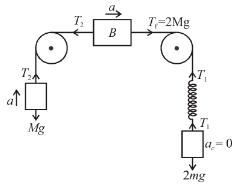Courses

# Newton's Law Of Motion NAT Level - 2

## 10 Questions MCQ Test Basic Physics for IIT JAM | Newton's Law Of Motion NAT Level - 2

Description
This mock test of Newton's Law Of Motion NAT Level - 2 for Physics helps you for every Physics entrance exam. This contains 10 Multiple Choice Questions for Physics Newton's Law Of Motion NAT Level - 2 (mcq) to study with solutions a complete question bank. The solved questions answers in this Newton's Law Of Motion NAT Level - 2 quiz give you a good mix of easy questions and tough questions. Physics students definitely take this Newton's Law Of Motion NAT Level - 2 exercise for a better result in the exam. You can find other Newton's Law Of Motion NAT Level - 2 extra questions, long questions & short questions for Physics on EduRev as well by searching above.
*Answer can only contain numeric values
QUESTION: 1

### In the figure shown blocks A and B are kept on a wedge C. A, B and C each have mass m. All surfaces are smooth. Find the acceleration of C.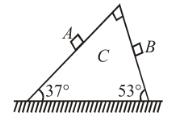Solution: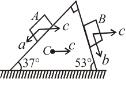Let c be acceleration of wedge C.
a be acceleration of block A w.r.t. wedge C.
b be acceleration of block B w.r.t. wedge C.
Applying Newton law in horizontal direction to system of A + B + C.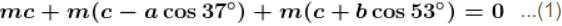Applying Newton's law of block A and B along the incline gives.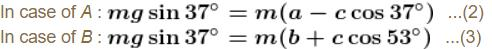Solving (1), (2) and (3)
we get c = 0
Acceleration of wedge C is 0

*Answer can only contain numeric values
QUESTION: 2

### System is shown in the figure. Assume that cylinder remains in contact with the two wedges. The velocity of cylinder  (in m/s) is :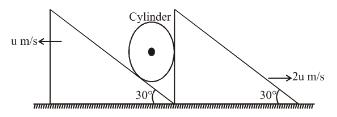Solution:

As cylinder will remain in contact with wedge A vx = 2u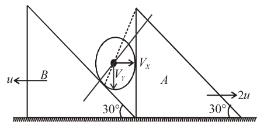As it also remain in contact with wedge B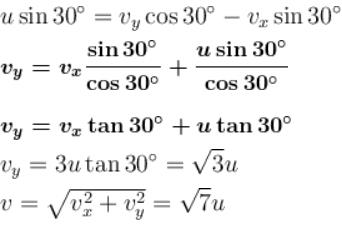*Answer can only contain numeric values
QUESTION: 3

### In the figure shown the velocity of lift is 2m/s while string is winding on the motor shaft with velocity 2m/s block A is moving downwards with a velocity of 2m/s, then find out the velocity of block B (in m/s).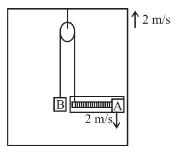Solution: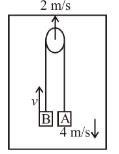v = velocity of B w.r.t. ground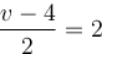v = 8 m/s    (velocity of B w.r.t. ground)
v = 8 m/s
v' =
6 m/s    (velocity of B w.r.t. lift)
v' = 6 m/s

*Answer can only contain numeric values
QUESTION: 4

In the arrangement shown, by what acceleration (in m/s2) the boy must go up so that 100kg block remains stationary on the wedge. The wedge is fixed and friction is absent everywhere. (Take g = 10 m/s2)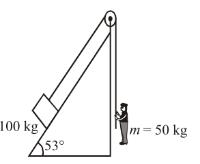Solution: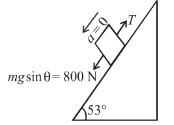For block to be stationary T = 800 N
If man moves up by acceleration ‘a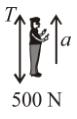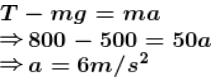*Answer can only contain numeric values
QUESTION: 5

In the figures shown P1 and P2 are massless pulleys. P1 is fixed and P2 can move. Masses of AB and C are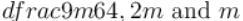respectively. All contacts are smooth and the string is massless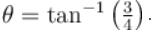Find the acceleration of block C in m/s2.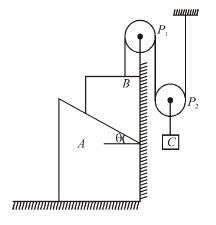Solution:

Let the acceleration of B downwards be aB = a
From constraint; acceleration of A and C are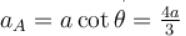towards left.
aC = a/2
free body diagram of AB and C are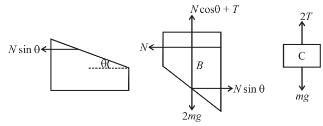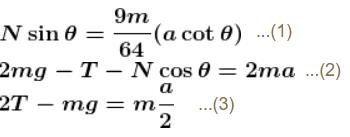solving we get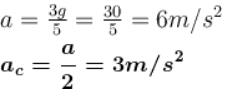*Answer can only contain numeric values
QUESTION: 6

In the arrangement shown in the Figure, a block of mass m = 2 kg  lies on a wedge on mass M = 8 kg. The initial acceleration of the wedge (if the surfaces are smooth) given by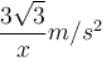then x is.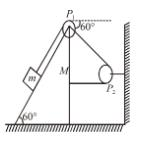Solution: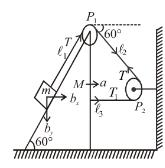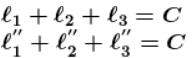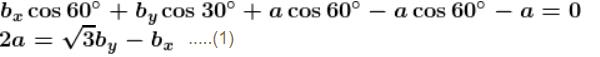from wedge constraint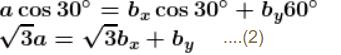Applying Newton’s law (wedge + block)

along horizontal direction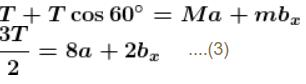Applying Newton’s law on block along the incline plane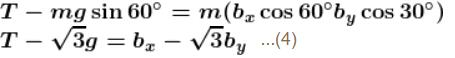Solving equation (1); (2); (3) and (4)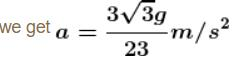*Answer can only contain numeric values
QUESTION: 7

Two block A and B each of mass m are placed on a smooth horizontal surface. Two horizontal force F and 2F are applied on both the blocks A and B respectively as shown in figure. The block A does not slide on block B. Then the normal reaction acting between the two blocks is nF : find the value of n.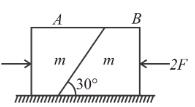Solution:

Acceleration of two mass system is a = F/2m leftward
FBD of block A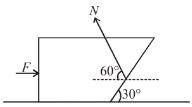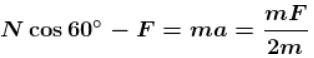solving N = 3F

*Answer can only contain numeric values
QUESTION: 8

In the figure shown, the pulleys and strings are massless. The acceleration (in m/s2) of the block of mass 4m just after the system is released from rest is ...........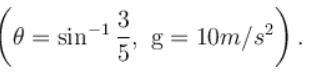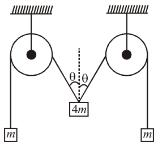Solution:

The FBD of block is as shown
From Newton’s second law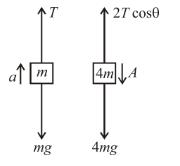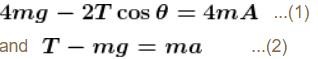cos θ = 4/5 and from constraint we get a = A cos θ ...(3)
solving equation (1), (2) and (3)
we get acceleration of block of mass 4m, a = 5g/11 downwards.

*Answer can only contain numeric values
QUESTION: 9

Inside a horizontally moving box, an experimenter finds that the when an object is placed on a smooth horizontal table and is released, it moves with an acceleration of 10 m/s2. In this box if 1 kg body is suspended with a light string, the tension (in N) in the string in equilibrium position. (w.r.t.) experimenter will be (Take g = 10 m/s2)

Solution:

Acceleration of box = 10 m/s2
Inside the box force acting on bob are shown in the figure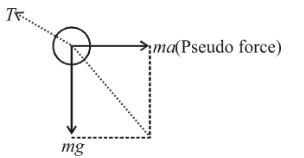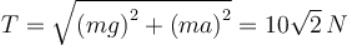*Answer can only contain numeric values
QUESTION: 10

In the figure shown all the contacts are smooth. Strings and spring and light. Initially A is held by someone and  B and C are at rest and in equilibrium also. Find out the acceleration of block C in m/s2 just after the block A is released. Masses of AB and C are MM and 2M respectively.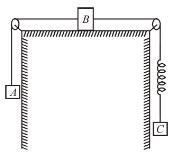Solution:

Before block A was released, the system was at rest, and all blocks were in equilibrium hence, tension in both the strings is equal to 2 Mg.
When block A is released, it will have an unbalanced force on it and hence the tension in string (2) will change to say T2. Now the arrangement will be
Since, tension in spring does not change instantaneously, hence, tension in string perpendicular will remain same i.e. 2Mg, Thus, Block C will remain at rest aC = 0.
Newton's law along the string (2),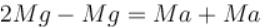⇒ a = g/2
Hence acceleration of A = g/2 upward, B = g/2 toward Right, and C = 0